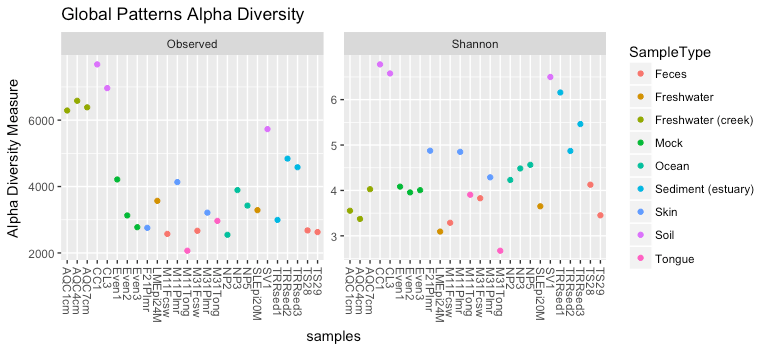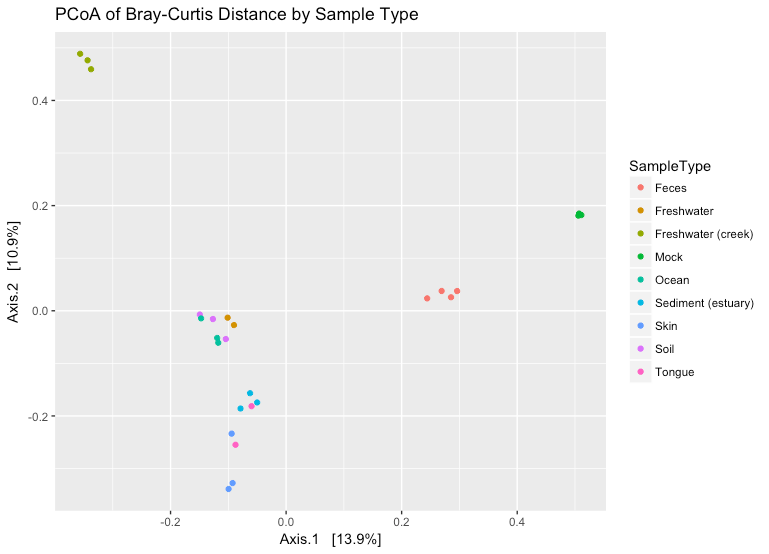During the second week we will spend a lot of time discussing the analysis of microbiome data. The following exercise was created to continue build upon the material provided in the ggplot2 lessons and provide some contextual examples of how the ggplot syntax is used for plotting microbial ecological data.

The PhyloSeq homepage provides additional vignettes which go beyond what is presented below.

Exercise 1: Alpha Diversity plots

Alpha diversity is a useful measure of the number of members and overall complexity of individual samples within a community. There are a large number of alpha diversity measures. These measures can be called upon in PhyloSeq and plotted using ggplot2 conventions.

For example, the plot below shows all available alpha diversity measures for the Global Patterns microbiome data set which is included as part of the PhyloSeq package. The command plot_richness is part of PhyloSeq. You can read more about it’s modifiers and capabilities using ?plot_richness.

First you will need to load the PhyloSeq library and initiate the data used in the exercise.

library(PhyloSeq)
data(GlobalPatterns)
plot_richness(GlobalPatterns)This set of plots is a lot of information. We can control this information using modifiers on plot_richness and ggplot2.### Alpha Diversity of Global Patterns

plot_richness(GlobalPatterns, title = “Global Patterns Alpha Diversity”, measures = c(“Observed”, “Shannon”), color = “SampleType”)

Since this is a ggplot2 object, it can be modified using ggplot2 functions. For example, you can use faceting to separate the data by Sample Type:### Global Patterns Alpha Diversity Faceted by Sample Type

plot_richness(GlobalPatterns, title = “Global Patterns Alpha Diversity”, measures = c(“Shannon”), color = “SampleType”) +
facet_wrap(~SampleType, scales = “free_x”)

Of note, in this case we used facet_wrap instead of facet_grid. Facet wrap automatically wraps the individual facets to your display window. Within facet_wrap we used scales=”free_x” which allows each facet to expand and contract ‘freely’ plotting only the data that are available for samples in that particular facet. You can turn this on and off to visualize the differences between plotting with and without a free_x.

Exercise 2: Ordination plots

In order to plot an ordination plot, you first need to perform the ordination. For example, if you want to visualize a PCoA plot of the Bray Curtis dissimilarity between all samples in the Global Patterns data you would do the following

GP.ord <- ordinate(GlobalPatterns, “PCoA”, “bray”)

plot_ordination(GlobalPatterns, GP.ord, type=”samples”, color = “SampleType”, title = “PCoA of Bray-Curtis Distance by Sample Type”)In the above sample we displayed the ordination for the samples. However, you can also visualize taxa ordination and use gpplot functionality to further interrogate your data.

Using the information learned today and the help page for plot_ordination, attempt to complete the following ordination plot:### Toggle Title

plot_ordination(GlobalPatterns, GP.ord, type=”taxa”, title = “PCoA of Bray-Curtis Distance by Sample Type”) + facet_wrap(~Phylum)